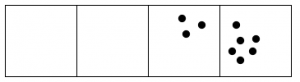$$\newcommand{\id}{\mathrm{id}}$$ $$\newcommand{\Span}{\mathrm{span}}$$ $$\newcommand{\kernel}{\mathrm{null}\,}$$ $$\newcommand{\range}{\mathrm{range}\,}$$ $$\newcommand{\RealPart}{\mathrm{Re}}$$ $$\newcommand{\ImaginaryPart}{\mathrm{Im}}$$ $$\newcommand{\Argument}{\mathrm{Arg}}$$ $$\newcommand{\norm}{\| #1 \|}$$ $$\newcommand{\inner}{\langle #1, #2 \rangle}$$ $$\newcommand{\Span}{\mathrm{span}}$$

# 2.2: Other Rules

•• Contributed by Michelle Manes
• Professor (Mathematics) at University of Hawaii
$$\newcommand{\vecs}{\overset { \rightharpoonup} {\mathbf{#1}} }$$ $$\newcommand{\vecd}{\overset{-\!-\!\rightharpoonup}{\vphantom{a}\smash {#1}}}$$$$\newcommand{\id}{\mathrm{id}}$$ $$\newcommand{\Span}{\mathrm{span}}$$ $$\newcommand{\kernel}{\mathrm{null}\,}$$ $$\newcommand{\range}{\mathrm{range}\,}$$ $$\newcommand{\RealPart}{\mathrm{Re}}$$ $$\newcommand{\ImaginaryPart}{\mathrm{Im}}$$ $$\newcommand{\Argument}{\mathrm{Arg}}$$ $$\newcommand{\norm}{\| #1 \|}$$ $$\newcommand{\inner}{\langle #1, #2 \rangle}$$ $$\newcommand{\Span}{\mathrm{span}}$$ $$\newcommand{\id}{\mathrm{id}}$$ $$\newcommand{\Span}{\mathrm{span}}$$ $$\newcommand{\kernel}{\mathrm{null}\,}$$ $$\newcommand{\range}{\mathrm{range}\,}$$ $$\newcommand{\RealPart}{\mathrm{Re}}$$ $$\newcommand{\ImaginaryPart}{\mathrm{Im}}$$ $$\newcommand{\Argument}{\mathrm{Arg}}$$ $$\newcommand{\norm}{\| #1 \|}$$ $$\newcommand{\inner}{\langle #1, #2 \rangle}$$ $$\newcommand{\Span}{\mathrm{span}}$$

Let’s play the dots and boxes game, but change the rule.

The 1←3 Rule

Whenever there are three dots in single box, they “explode,” disappear, and become one dot in the box to the left.

Example $$\PageIndex{1}$$: Fifteen dots in the 1←3 system

Here’s what happens with fifteen dots:The 1←3 code for fifteen dots is: 120.

Problem 2

1. Show that the 1←3 code for twenty dots is 202.
2. What is the 1←3 code for thirteen dots?
3. What is the 1←3 code for twenty-five dots?
4. What number of dots has 1←3 code 1022?
5. Is it possible for a collection of dots to have 1←3 code 2031? Explain your answer.

Problem 3

1. Describe how the 1←4 rule would work.
2. What is the 1←4 code for thirteen dots?

Problem 4

1. What is the 1←5 code for the thirteen dots?
2. What is the 1←5 code for five dots?

Problem 5

1. What is the 1←9 code for thirteen dots?
2. What is the 1←9 code for thirty dots?

Problem 6

1. What is the 1←10 code for thirteen dots?
2. What is the 1←10 code for thirty-seven dots?
3. What is the 1←10 code for two hundred thirty-eight dots?
4. What is the 1←10 code for five thousand eight hundred and thirty-three dots?

Think / Pair / Share

After you have worked on the problems on your own, compare your ideas with a partner. Can you describe what’s going on in Problem 6 and why?